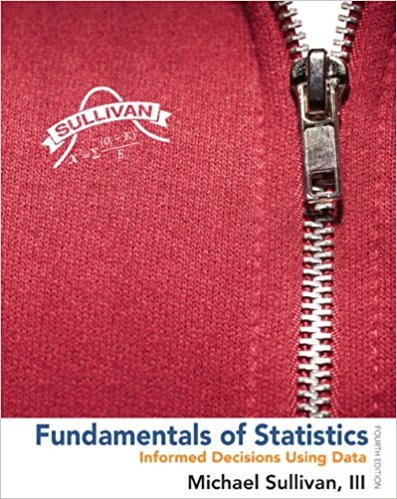×
×

# Solved: SMART Car The following data represent the milesISBN: 9780321838704 30

## Solution for problem 21AYU Chapter Chapter 3.4

Fundamentals of Statistics | 4th Edition

• Textbook Solutions
• 2901 Step-by-step solutions solved by professors and subject experts
• Get 24/7 help from StudySoup virtual teaching assistantsFundamentals of Statistics | 4th Edition

4 5 1 276 Reviews
19
0
Problem 21AYU

Problem

SMART Car The following data represent the miles per gallon of a random sample of SMART cars with a threecylinder, 1.0-liter engine.

 31.5 36.0 37.8 38.4 40.1 42.3 34.3 36.3 37.9 38.8 40.6 42.7 34.5 37.4 38.0 39.3 41.4 43.5 35.5 37.5 38.3 39.5 41.5 47.5 Source: www.fueleconomy.gov

(a) Compute the z-score corresponding to the individual who obtained 36.3 miles per gallon. Interpret this result.

(b) Determine the quartiles.

(c) Compute and interpret the interquartile range, IQR.

(d) Determine the lower and upper fences. Are there any outliers?

Step-by-Step Solution:

Step 1 :

Given, the miles per gallon of a random sample of SMART cars with a three cylinder, 1.0-liter engine.

 31.5 36 37.8 38.4 40.1 42.3 34.3 36.3 37.9 38.8 40.6 42.7 34.5 37.4 38 39.3 41.4 43.5 35.5 37.5 38.3 39.5 41.5 47.5
We have to compute the z-score corresponding to the individual who obtained 36.3 miles per gallonMeanand=3.41

The Test Statistic is given byZ = -0.73

b) Quartiles

We have n = 24, so for lower quartile we have to add 6th and 7th value and divide it by 2=...

Step 2 of 3

Step 3 of 3

##### ISBN: 9780321838704

This textbook survival guide was created for the textbook: Fundamentals of Statistics, edition: 4. Fundamentals of Statistics was written by and is associated to the ISBN: 9780321838704. This full solution covers the following key subjects: compute, smart, interpret, gallon, determine. This expansive textbook survival guide covers 51 chapters, and 2146 solutions. The answer to “SMART Car The following data represent the miles per gallon of a random sample of SMART cars with a threecylinder, 1.0-liter engine.31.536.037.838.440.142.334.336.337.938.840.642.734.537.438.039.341.443.535.537.538.339.541.547.5Source: www.fueleconomy.gov(a) Compute the z-score corresponding to the individual who obtained 36.3 miles per gallon. Interpret this result.________________(b) Determine the quartiles.________________(c) Compute and interpret the interquartile range, IQR.________________(d) Determine the lower and upper fences. Are there any outliers?” is broken down into a number of easy to follow steps, and 59 words. The full step-by-step solution to problem: 21AYU from chapter: Chapter 3.4 was answered by , our top Statistics solution expert on 04/03/17, 08:02AM. Since the solution to 21AYU from Chapter 3.4 chapter was answered, more than 5952 students have viewed the full step-by-step answer.

Unlock Textbook Solution